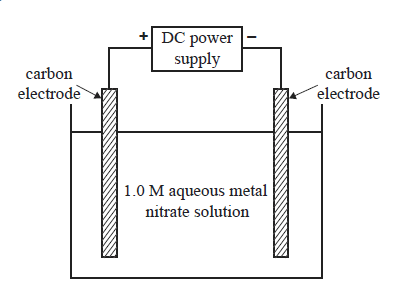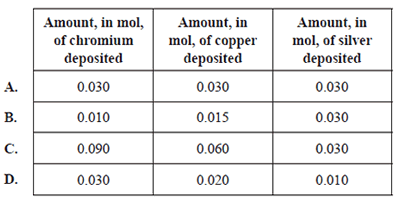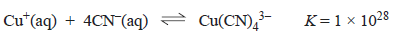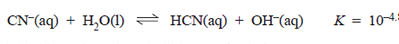Electrochemistry (2011 VCE)-galvanic cells 1) A series of electrolysis experiments is conducted using the apparatus shown below.An electric charge of 0.030 faraday was passed through separate solutions of 1.0 Cr(NO3 )3, 1.0 M Cu(NO3 )2 and 1.0 M AgNO3 . In each case the corresponding metal was deposited on the negative electrode. The amount in mol of each metal deposited isSolution. 2) An ornament was coated with a metal, M, by electrolysis of a solution of the metal ion, Mx+ . During the electrolysis a current of 1.50 amperes was applied for 180 seconds. The ornament was coated in 0.0014 mol of metal. The value of x in Mx+ a) 1 b) 2 c) 3 d) 4 Solution3) A chemical engineer designs a pilot plant to determine the conditions that will give the best results for copper plating different objects. A range of experiments indicates that an electroplating cell with an aqueous electrolyte containing copper(I)cyanide, CuCN, potassium cyanide, KCN, and potassium hydroxide, KOH, will produce a uniform copper coating. i) Write a balanced half equation for the cathode reaction in this electrolytic cell. Solutionii) The quality of the copper coating depends on maintaining a low concentration of copper(I) ions in the electrolyte. This is achieved by making use of the following reaction which takes place in the electrolyte bath. In this reaction, copper(I) ions, Cu+.react with cyanide ions CN- according to the equation .Refer to this information to explain how the presence of excess potassium cyanide in the electrolyte maintains a low concentration of Cu+ (aq) ions in solution. Solutioniii) The cyanide ion, CN- , is the conjugate base of the acid hydrogen cyanide, HCN.Hydrogen cyanide is highly toxic and can bubble out of solution. Explain how the presence of potassium hydroxide in the electrolyte is essential to the safe operation of this cell. Solutioniv) Any gas produced at the cathode is found to damage the quality of the copper plate. This is avoided by maintaining a low current. Write a balanced equation for the gas produced at the cathode if the current is too high. Solutionv) In one trial, a medal is copper plated in the cell. The experimental data is given below. Mass of medal before copper plating = 25.2 g Mass of medal after copper plating =36.4 g Current = 0.900 A Calculate, the time, in minutes taken to copper plate the medal. Solution